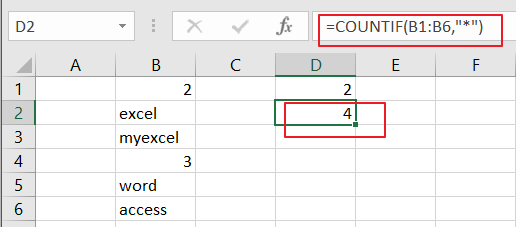# How to Count Cells that Contain Text in Excel

This post will guide you how to count the number of cells that text only within a range of cells using a formula in Excel 2013/2016 or Excel office 365. How do I count the number of cells with text values(not errors, not numbers, not blanks) using a simple formula in Excel. When you analyze a large list of data with texts and numbers, you may also want to know that how many cells that contain text values. The below steps will show you how to do it in a simple way.

## Count Number of Cells that Contain Text

You can use the COUNTIF function to count cells that contain text (not errors, not numbers, not blanks). And you can use the COUNTIF function with a wildcard that matches any number of characters in cells.

The below is a generic formula to count the number of cells containing text:

=COUNTIF(range,*)

Assuming that you have a list of data in range B1:B6 and you want to count the number of cells with text values only. And you can add the the asterisk(*) as a wildcard in the second argument as its criteria and you will get the below formula:

=COUNTIF(B1:B6,”*”)LET’S SEE THAT HOW THIS FORMULA WORKS:

The COUNTIF function can be used to count the number of cells that match a single condition or criteria. And in this case, you need to provide a criteria as “*”, which is evaluated as “values that containing any text characters”. Then the total count of all cells in the range B1:B6 that meet the above criteria is returned.

If you want to count cells with text characters in a range of cells, but exclude cells with only a space character, and you can use the COUNTIFS function to supply more specific criteria to achieve the result. Using the below generic formula:

=COUNTIFS(B1:B6,”*”,B1:B6,”<> “)

### Related Functions

• Excel COUNTIF function
The Excel COUNTIF function will count the number of cells in a range that meet a given criteria. This function can be used to count the different kinds of cells with number, date, text values, blank, non-blanks, or containing specific characters. etc. = COUNTIF (range, criteria) …
• Excel COUNTIFS function
The Excel COUNTIFS function returns the count of cells in a range that meet one or more criteria. The syntax of the COUNTIFS function is as below:= COUNTIFS(criteria_range1, criteria1, [criteria_range2, criteria2]…)…